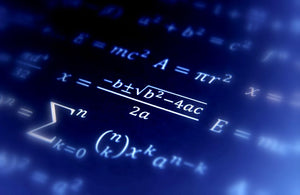Start Your Journey with ICS--Enroll Today!# College Algebra

Regular price
\$399.00
Sale price
\$399.00
Regular price
\$1,044.00
Sold out
Unit price
per

## Course Description

This course covers a broad survey of key concepts of college algebra. Students will be introduced to solving equations and inequalities, functions (including linear, quadratic, polynomial, rational, exponential, and logarithmic), systems of equations and inequalities, and series and sequences.

## Course Outcomes

At the end of this course, you will be able to:

• Differentiate among various processes used in manipulating algebraic expressions
• Analyze algebraic equations and inequalities
• Evaluate a function
• Evaluate the relationship between functions and graphs illustrating functions
• Analyze polynomial and rational functions
• Evaluate exponential and logarithmic functions
• Apply various techniques to solve systems of linear equations and inequalities
• Evaluate sequences and series
• Analyze the factorial function

## Course MaterialsTitle: Edition: Author: ISBN: College Algebra7Blitzer9780134469164